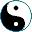We are in Part category. Take an set A, and let's define the following functor: PlusNull: X ↦ (X+Null) We already talked about this functor, and last time it was from Part to Set. This time we composed it with the inclusion of Set to Part, thus getting an endofunctor. Why is it a monad? We need uX: X → (X+Null) and mX: ((X+Null) + Null)→ (X + Null) . The first one is a simple inclusion; the second one maps both Null singletons to Null. In Lisp it looks like this: ```(define (ux x) x) (define (mx x) x)``` As you see, it is a monad (if you don't, prove it as an exercise).